# How to extract substrings in Excel using regular expressions (Regex)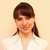by , updated on

In this tutorial, you'll learn how to use regular expressions in Excel to find and extract substrings matching a given pattern.

Microsoft Excel provides a number of functions to extract text from cells. Those functions can cope with most of string extraction challenges in your worksheets. Most, but not all. When the Text functions stumble, regular expressions come to rescue. Wait… Excel has no RegEx functions! True, no inbuilt functions. But there's nothing that would prevent you from using your own ones :)

## Excel VBA Regex function to extract strings

To add a custom Regex Extract function to your Excel, paste the following code in the VBA editor. In order to enable regular expressions in VBA, we are using the built-in Microsoft RegExp object.

If you have little experience with VBA, a step-by-step user guide may prove helpful: How to insert VBA code in Excel.

Note. For the function to work, be sure to save your file as a macro-enabled workbook (.xlsm).

### RegExpExtract syntax

The RegExpExtract function searches an input string for values that match a regular expression and extracts one or all matches.

The function has the following syntax:

RegExpExtract(text, pattern, [instance_num], [match_case])

Where:

• Text (required) - the text string to search in.
• Pattern (required) - the regular expression to match. When supplied directly in a formula, the pattern should be enclosed in double quotation marks.
• Instance_num (optional) - a serial number that indicates which instance to extract. If omitted, returns all found matches (default).
• Match_case (optional) - defines whether to match or ignore text case. If TRUE or omitted (default), case-sensitive matching is performed; if FALSE - case-insensitive.

The function works in all versions of Excel 365, Excel 2021, Excel 2019, Excel 2016, Excel 2013 and Excel 2010.

### 4 things you should know about RegExpExtract

To effectively use the function in your Excel, there are a few important things to take notice of:

1. By default, the function returns all found matches into neighboring cells like shown in this example. To get a specific occurrence, supply a corresponding number to the instance_num argument.
2. By default, the function is case-sensitive. For case-insensitive matching, set the match_case argument to FALSE. Because of the VBA limitations, the case-insensitive construct (?i) won't work.
3. If a valid pattern is not found, the function returns nothing (empty string).
4. If the pattern is invalid, a #VALUE! error occurs.

Before you start using this custom function in your worksheets, you need to understand what it is capable of, right? The below examples cover a few common use cases and explain why the behavior may differ in Dynamic Array Excel (Microsoft 365 and Excel 2021) and traditional Excel (2019 and older versions).

Note. Out regex examples are written for fairy simple data sets. We cannot guarantee they will work flawlessly in your real worksheets. Those who have experience with regex would agree that writing regular expressions is a never-ending road to perfection - almost always there's a way to make it more elegant or capable of handling a wider range of input data.

### Regex to extract number from string

Following the basic maxim of teaching "from simple to complex", we'll start with a very plain case: extracting number from string.

The first thing for you to decide is which number to retrieve: first, last, specific occurrence or all numbers.

#### Extract first number

This is as simple as regex can get. Given that \d means any digit from 0 to 9, and + means one or more times, our regular expression takes this form:

Pattern: \d+

Set instance_num to 1 and you'll get the desired result:

`=RegExpExtract(A5, "\d+", 1)`

Where A5 is the original string.

For convenience, you can input the pattern in a predefined cell (\$A\$2 ) and lock its address with the \$ sign:

`=RegExpExtract(A5, \$A\$2, 1)`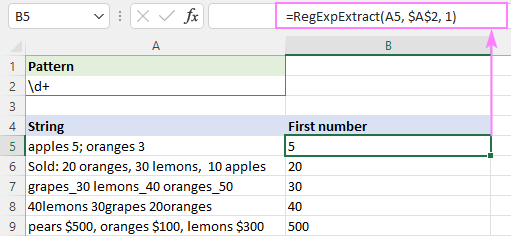#### Get last number

To extract the last number in a string, here's the pattern to use:

Pattern: (\d+)(?!.*\d)

Translated into a human language, it says: find a number that is not followed (anywhere, not just immediately) by any other number. To express this, we are using a negative lookahead (?!.*\d), which means that to the right of the pattern there should be no other digit (\d) regardless of how many other characters are before it.

`=RegExpExtract(A5, "(\d+)(?!.*\d)")`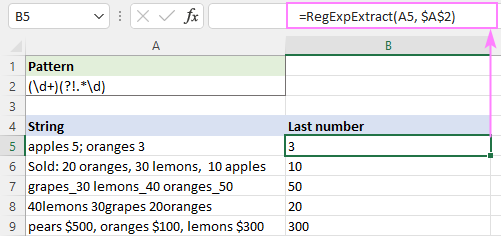Tips:

• To get a specific occurrence, use \d+ for pattern and an appropriate serial number for instance_num.
• The formula to extract all numbers is discussed in the next example.

### Regex to extract all matches

Pushing our example a little further, suppose you want to get all numbers from a string, not just one.

As you may remember, the number of extracted matches is controlled by the optional instance_num argument. The default is all matches, so you simply omit this parameter:

`=RegExpExtract(A2, "\d+")`

The formula works beautifully for a single cell, but the behavior differs in Dynamic Array Excel and non-dynamic versions.

#### Excel 365 and Excel 2021

Due to support for dynamic arrays, a regular formula automatically spills into as many cells as needed to display all calculated results. In terms of Excel, this is called a spilled range: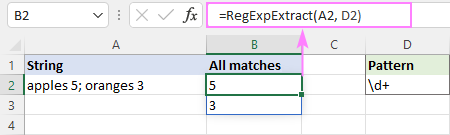#### Excel 2019 and lower

In pre-dynamic Excel, the above formula would return just one match. To get multiple matches, you need to make it an array formula. For this, select a range of cells, type the formula, and press Ctrl + Shift + Enter to complete it.

A downside of this approach is a bunch of #N/A errors appearing in "extra cells". Regrettably, nothing can be done about it (neither IFERROR nor IFNA can fix it, alas).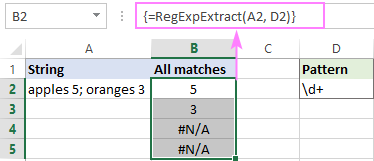#### Extract all matches in one cell

When processing a column of data, the above approach obviously won't work. In this case, an ideal solution would be returning all matches in a single cell. To have it done, serve the results of RegExpExtract to the TEXTJOIN function and separate them with any delimiter you like, say a comma and a space:

`=TEXTJOIN(", ", TRUE, RegExpExtract(A5, "\d+"))`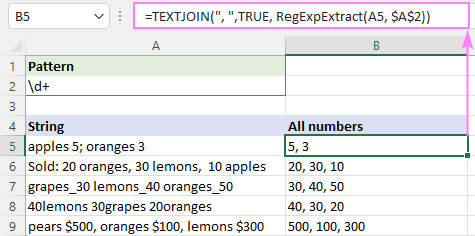Note. Because the TEXTJOIN function is only available in Excel for Microsoft 365, Excel 2021 and Excel 2019, the formula won't work in older versions.

### Regex to extract text from string

Extracting text from an alphanumeric string is quite a challenging task in Excel. With regex, it becomes as easy as pie. Just use a negated class to match everything that is not a digit.

Pattern: [^\d]+

To get substrings in individual cells (spill range), the formula is:

`=RegExpExtract(A5, "[^\d]+")`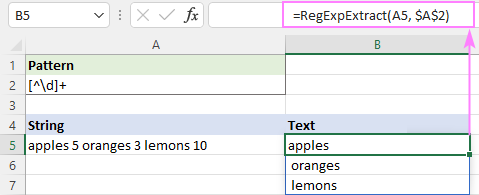To output all matches into one cell, nest the RegExpExtract function in TEXTJOIN like this:

`=TEXTJOIN("", TRUE, RegExpExtract(A5, "[^\d]+"))`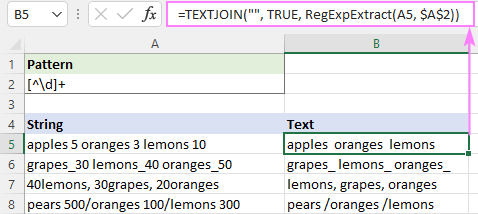### Regex to extract email address from string

To pull out an email address from a string containing a lot of different information, write a regular expression that replicates the email address structure.

Pattern: [\w\.\-]+@[A-Za-z0-9\.\-]+\.[A-Za-z]{2,24}

Breaking down this regex, here's what we get:

• [\w\.\-]+ is a username that may include 1 or more alphanumeric characters, underscores, dots and hyphens.
• @ symbol
• [A-Za-z0-9\.\-]+ is a domain name consisting of: uppercase and lowercase letters, digits, hyphens and dots (in case of subdomains). Underscores are not allowed here, therefore 3 different character sets (such as A-Z a-z and 0-9) are used instead of \w that matches any letter, digit or underscore.
• \.[A-Za-z]{2,24} is a top-level domain. Consists of a dot followed by uppercase and lowercase letters. Most of top-level domains are 3-letters long (e.g .com .org, .edu, etc.), but in theory it can contain from 2 to 24 letters (the longest registered TLD).

Assuming the string is in A5 and the pattern in A2, the formula to extract an email address is:

`=RegExpExtract(A5, \$A\$2)`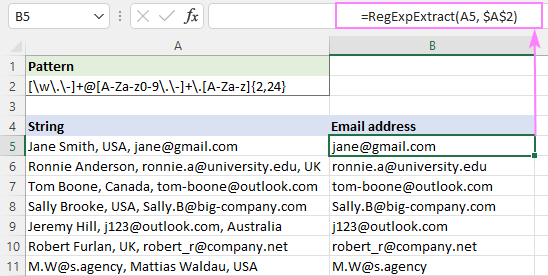### Regex to extract domain from email

When it comes to extracting email domain, the first thought that comes to mind is using a capturing group to find text that immediately follows the @ character.

Pattern: @([A-Za-z0-9\.\-]+\.[A-Za-z]{2,24})

Serve it to our RegExp function:

`=RegExpExtract(A5, "@([A-Za-z0-9\.\-]+\.[A-Za-z]{2,24})")`

And you'll get this result: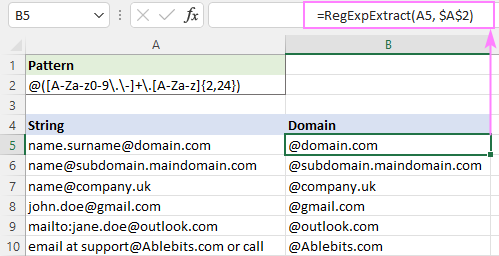With classic regular expressions, anything outside a capturing group is not included in the extraction. No one knows why VBA RegEx works differently and captures "@" as well. To get rid of it, you can remove the first character from the result by replacing it with an empty string.

`=REPLACE(RegExpExtract(A5, "@([a-z\d][a-z\d\-\.]*\.[a-z]{2,})", 1, FALSE), 1, 1, "")`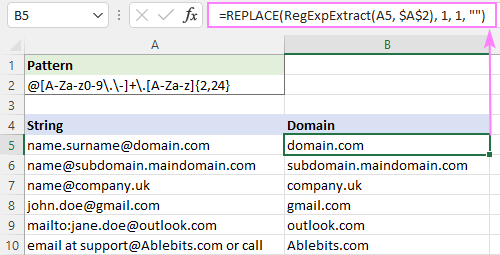### Regular expression to extract phone numbers

Phone numbers can be written in many different ways, which is why it's next to impossible to come up with a solution working under all circumstances. Nevertheless, you can write down all the formats used in your dataset and try to match them.

For this example, we are going to create a regex that will extract phone numbers in any of these formats:

 (123) 345-6789 (123) 345 6789 (123)3456789 123-345-6789 123.345.6789 123 345 6789 1233456789

Pattern: \(?\d{3}[-\. \)]*\d{3}[-\. ]?\d{4}\b

• The first part \(?\d{3} matches zero or one opening parenthesis followed by three digits d{3}.
• The [-\. \)]* part means any character in square brackets appearing 0 or more times: hyphen, period, space or closing parenthesis.
• Next, we have three digits again d{3} followed by any hyphen, period or space [-\. ]? appearing 0 or 1 time.
• After that, there is a group of four digits \d{4}.
• Finally, there is a word boundary \b defining that a phone number we are looking for cannot be part of a bigger number.

The complete formula takes this shape:

`=RegExpExtract(A5, "\(?\d{3}[-\. \)]*\d{3}[-\. ]?\d{4}\b")`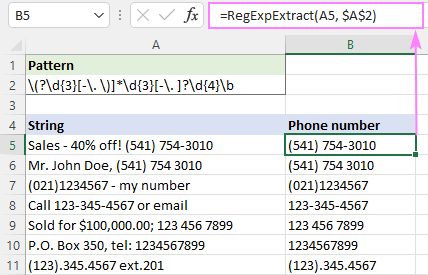Please be aware that the above regex may return a few false-positive results such as 123) 456 7899 or (123 456 7899. The below version fixes these issues. However, this syntax only works in VBA RegExp functions, not in classic regular expressions.

Pattern: (\(\d{3}\)|\d{3})[-\. ]?\d{3}[-\. ]?\d{4}\b

### Regex to extract date from string

A regular expression for extracting a date depends on the format in which the date appears within a string. For example:

To extract dates like 1/1/21 or 01/01/2021, the regex is: \d{1,2}\/\d{1,2}\/(\d{4}|\d{2})

It searches for a group of 1 or 2 digits d{1,2} followed by a slash, followed by another group of 1 or 2 digits, followed by a slash, followed by a group of 4 or 2 digits (\d{4}|\d{2}). Please notice that we look for 4-digit years first, and only then for 2-digit years. If we write it the other way around, only the first 2 digits will be picked out from all the years. This is because after the first condition is in the alternation OR construct is matched, the remaining conditions are not checked.

To retrieve dates like 1-Jan-21 or 01-Jan-2021, the pattern is: \d{1,2}-[A-Za-z]{3}-\d{2,4}

It searches for a group of 1 or 2 digits, followed by a hyphen, followed by a group of 3 uppercase or lowercase letters, followed by a hyphen, followed by a group of 4 or 2 digits.

After combining the two patterns together, we get the following regex:

Pattern: \b\d{1,2}[\/-](\d{1,2}|[A-Za-z]{3})[\/-](\d{4}|\d{2})\b

Where:

• The first part is 1 or 2 digits: \d{1,2}
• The second part is either 1 or 2 digits or 3 letters: (\d{1,2}|[A-Za-z]{3})
• The third part is a group of 4 or 2 digits: (\d{4}|\d{2})
• Delimiter is either a forward slash or hyphen: [\/-]
• A word boundary \b is placed on both sides to make it clear that a date is a separate word, and not part of a bigger string.

As you can see in the image below, it successfully pulls out dates and leaves out substrings such as 11/22/333. However, it still returns false positive results. In our case, the substring 11-ABC-2222 in A9 technically matches the date format dd-mmm-yyyy and therefore is extracted.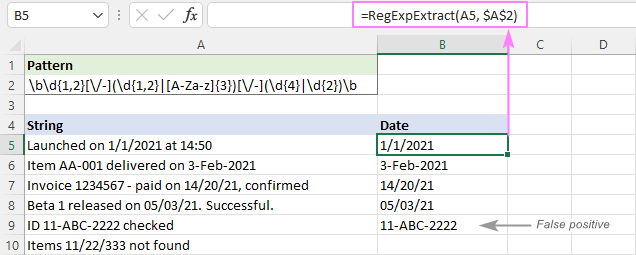To eliminate false positives, you can replace the [A-Za-z]{3} part with a full list of 3-letter month abbreviations:

Pattern: \b\d{1,2}[\/-](\d{1,2}|(Jan|Feb|Mar|Apr|May|Jun|Jul|Aug|Sep|Oct|Nov|Dec))[\/-](\d{4}|\d{2})\b

To ignore the letter case, we set the last argument of our custom function to FALSE:

`=RegExpExtract(A5, \$A\$2, 1, FALSE)`

And this time, we get a perfect result: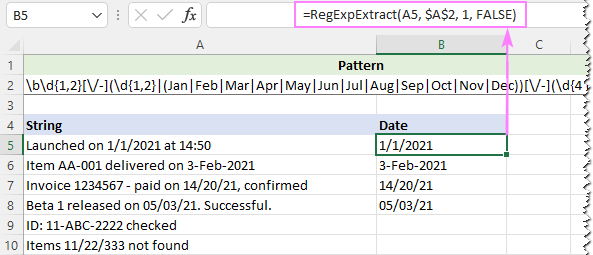### Regex to extract time from string

To get time in the hh:mm or hh:mm:ss format, the following expression will work a treat.

Pattern: \b(0?[0-9]|1[0-2]):[0-5]\d(:[0-5]\d)?\s?(AM|PM)\b|\b([0-9]|[0-1]\d|2[0-3]):[0-5]\d(:[0-5]\d)?(?!:)

Breaking down this regex, you can see 2 parts separated by | that enables the OR logic. In other words, we search for a substring that matches one of the below expressions.

Expression 1: \b(0?[0-9]|1[0-2]):[0-5]\d(:[0-5]\d)?\s?(AM|PM)\b

Retrieves times with AM/PM.

Hour can be any number from 0 to 12. To get it, we use the OR construct ([0-9]|1[0-2]), where:

• [0-9] matches any number from 0 to 9
• 1[0-2] matches any number from 10 to 12

Minute [0-5]\d is any number from 00 to 59.

Second (:[0-5]\d)? is also any number from 00 to 59. The ? quantifier means zero or one occurrence since seconds may or may not be included in the time value.

Expression 2: \b([0-9]|[0-1]\d|2[0-3]):[0-5]\d(:[0-5]\d)?(?!:)

Extracts times without AM/PM.

The hour part can be any number from 0 to 32. To get it, a different OR construct ([0-9]|[0-1]\d|2[0-3]) is used, where:

• [0-9] matches any number from 0 to 9
• [0-1]\d matches any number from 00 to 19
• 2[0-3] matches any number from 20 to 23

The minute and second parts are the same as in expression 1 above.

The negative lookahead (?!:) is added to skip strings such as 20:30:80.

As PM/AM can be either uppercase or lowercase, we make the function case-insensitive:

`=RegExpExtract(A5, \$A\$2, 1, FALSE)`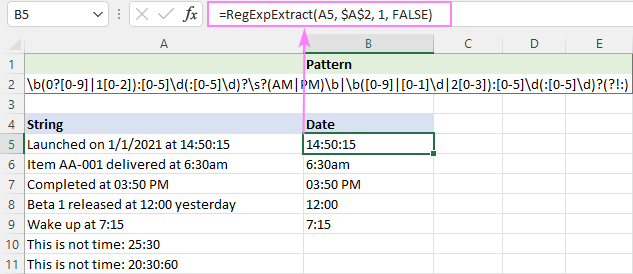Hopefully, the above examples gave you some ideas on how to use regular expressions in your Excel worksheets. Unfortunately, not all features of classic regular expressions are supported in VBA. If your task cannot be accomplished with VBA RegExp, I encourage you to read the next part that discusses a lot more powerful .NET Regex functions.

## Custom .NET based Regex function to extract text in Excel

Unlike VBA RegExp functions that can be written by any Excel user, .NET RegEx is the developer's realm. The Microsoft .NET Framework supports full-featured regular expression syntax compatible with Perl 5. This article won't teach you how to write such functions (I am not a programmer and don't have a slightest idea on how to do that :)

Four powerful functions processed by the standard .NET RegEx engine are already written by our developers and included in Ultimate Suite. Below, we'll demonstrate some practical uses of the function specially designed for extracting text in Excel.

Tip. For information about the .NET Regex syntax, please refer to .NET Regular Expression Language.

### How to extract stings in Excel using regular expressions

Assuming you have the latest version of Ultimate Suite installed, extracting text using regular expressions boils down to these two steps:

1. On the Ablebits Data tab, in the Text group, click Regex Tools.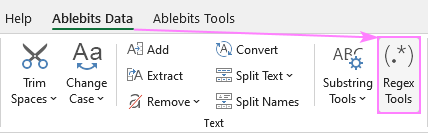2. On the Regex Tools pane, select the source data, enter your Regex pattern, and choose the Extract option. To get the result as a custom function, not a value, select the Insert as a formula check box. When done, click the Extract button.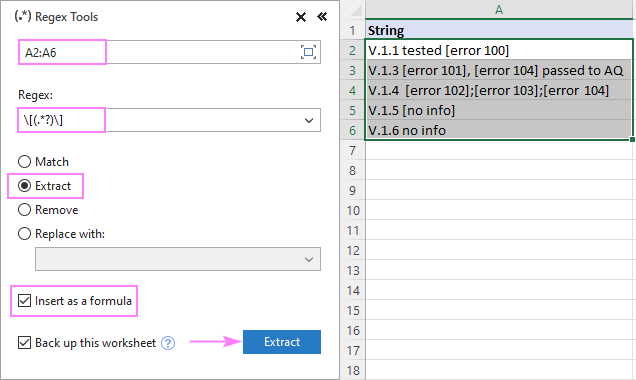The results will appear in a new column to the right of your original data: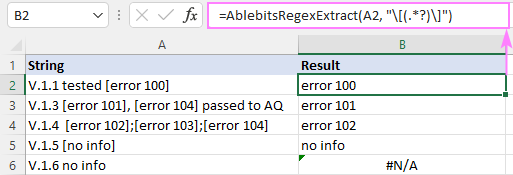### AblebitsRegexExtract syntax

Our custom function has the following syntax:

AblebitsRegexExtract(reference, regular_expression)

Where:

• Reference (required) - a reference to the cell containing the source string.
• Regular_expression (required) - the regex pattern to match.

Important note! The function only works on the machines with Ultimate Suite for Excel installed.

### Usage notes

To make your learning curve smoother and your experience more enjoyable, please pay attention to these points:

1. To create a formula, you can use our Regex Tools, or Excel's Insert function dialog, or type the full function name in a cell. Once the formula is inserted, you can manage it (edit, copy or move) like any native formula.
2. The pattern you enter on the Regex Tools pane goes to the 2nd argument. It is also possible to keep a regular expression in a separate cell. In this case, just use a cell reference for the 2nd argument.
3. The function extracts the first found match.
4. By default, the function is case-sensitive. For case-insensitive matching, use the (?i) pattern.
5. If a match is not found, an #N/A error is returned.

### Regex to extract string between two characters

To get text between two characters, you can use either a capturing group or look-arounds.

Let's say you are looking to extract text between brackets. A capturing group is the easiest way.

Pattern 1: \[(.*?)\]

With a positive lookbehind and lookahead, the result will be exactly the same.

Pattern 2: (?<=\[)(.*?)(?=\])

Please pay attention that our capturing group (.*?) performs a lazy search for text between two brackets - from the first [ to the first ]. A capturing group without a question mark (.*) would do a greedy search and capture everything from the first [ to the last ].

With the pattern in A2, the formula goes as follows:

`=AblebitsRegexExtract(A5, \$A\$2)`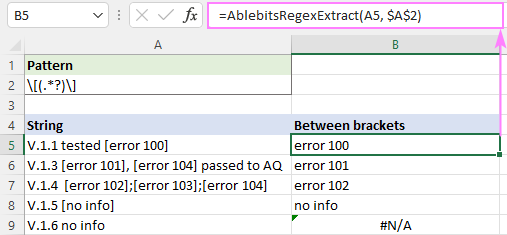#### How to get all matches

As already mentioned, the AblebitsRegexExtract function can only extract one match. To get all matches, you can use the VBA function we've discussed earlier. However, there is one caveat - VBA RegExp does not support capturing groups, so the above pattern will return the "boundary" characters as well, brackets in our case.

`=TEXTJOIN(" ", TRUE, RegExpExtract(A5, \$A\$2))`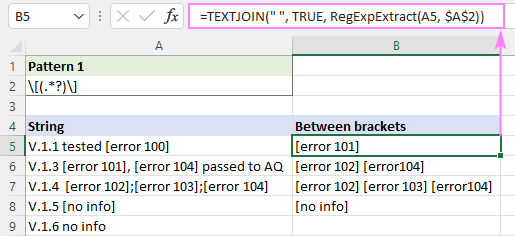To get rid of the brackets, SUBSTITUTE them with empty strings ("") using this formula:

`=SUBSTITUTE(SUBSTITUTE(TEXTJOIN(", ", TRUE, RegExpExtract(A5, \$A\$2)), "]", ""),"[","")`

For better readability, we are using a comma for delimiter.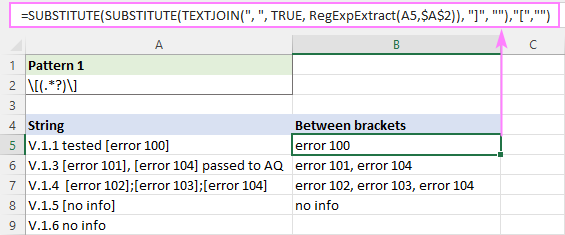### Regex to extract text between two strings

The approach we've worked out for pulling out text between two characters will also work for extracting text between two strings.

For example, to get everything between "test 1" and "test 2", use the following regular expression.

Pattern: test 1(.*?)test 2

The complete formula is:

`=AblebitsRegexExtract(A5, "test 1(.*?)test 2")`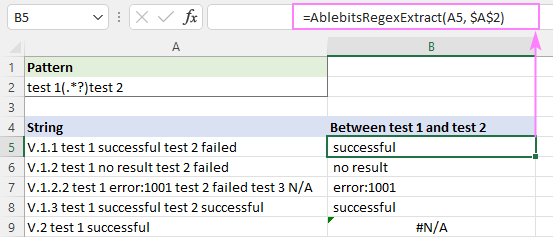### Regex to extract domain from URL

Even with regular expressions, extracting domain names from URLs is not a trivial task. The key element that does the trick is non-capturing groups. Depending on your ultimate goal, choose one of the below regexes.

To get a full domain name including subdomains

Pattern: (?:https?\:|^|\s)\/\/((?:[A-Za-z\d\-\.]{2,255}\.)?[A-Za-z\d\-]{1,63}\.[A-Za-z]{2,24})

To get a second-level domain without subdomains

Pattern: (?:https?\:|^|\s)\/\/(?:[A-Za-z\d\-\.]{2,255}\.)?([A-Za-z\d\-]{1,63}\.[A-Za-z]{2,24})

Now, let's see how these regular expressions work on an example of "https://www.mobile.ablebits.com" as a sample URL:

• (?:https?\:|^|\s) - non-capturing group. It matches but does not capture a substring that is preceded by one of the following: https, http, start of string (^), a whitespace character (\s). The last two items are included to handle protocol-relative URLs like "//google.com".
• \/\/ - two forward slashes (each is preceded by a backslash to escape the special meaning of the forward slash and interpret it literally).
• (?:[A-Za-z\d\-\.]{2,255}\.)? - non-capturing group to identify third-level, fourth-level, etc. domains, if any (mobile in our sample URL). In the first pattern, it is placed within a bigger capturing group to get all such subdomains included into the extraction. A subdomain can be from 2 to 255 characters long, hence the {2,255} quantifier.
• ([A-Za-z\d\-]{1,63}\.[A-Za-z]{2,24}) - capturing group to extract the second-level domain (ablebits) and the top-level domain (com). The max length of a second-level domain is 63 characters. The longest top-level domain currently in existence contains 24 characters.

Depending on which regular expression is entered in A2, the below formula will produce different results:

`=AblebitsRegexExtract(A5, \$A\$2)`

Regex to extract the full domain name with all subdomains: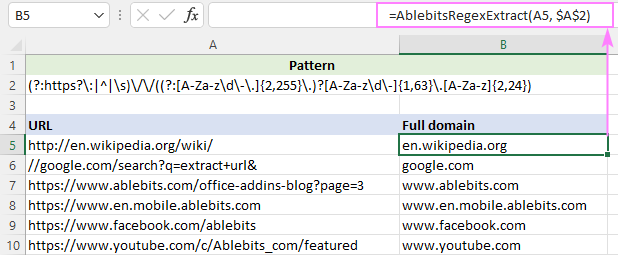Regex to extract a second-level domain without subdomains: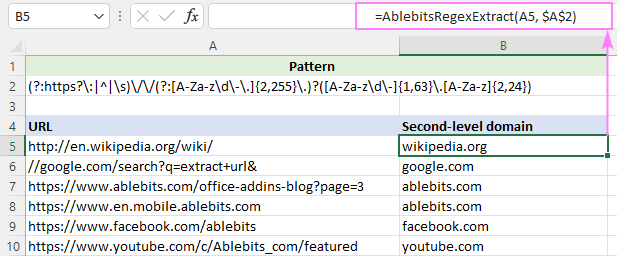That's how to extract parts of text in Excel using regular expressions. I thank you for reading and look forward to seeing you on our blog next week!